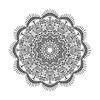## Welcome to the Treehouse Community

Want to collaborate on code errors? Have bugs you need feedback on? Looking for an extra set of eyes on your latest project? Get support with fellow developers, designers, and programmers of all backgrounds and skill levels here with the Treehouse Community!

### Looking to learn something new?

Treehouse offers a seven day free trial for new students. Get access to thousands of hours of content and join thousands of Treehouse students and alumni in the community today.# Consideration on "guess = getRandomNumber(upper)"

Hi everyone, I'm trying to learn what happens when this statement encounter but it's not so clear. Can someone make an example with numbers pls? I do not understand if, when this one encounter, guess is assigned a value generated from calling again the function, when enter in the loop. I mean.. when I arrive at this point, the function is called and the number is assigned to guess? Can you make an example with numbers from the beginning? Thanks to all!

We'll walk through the code:

```//create a variable named upper and give it the value 10000
var upper = 10000;

//call the getRandomNumber() function with upper as the actual parameter,
//and assign the returned value to a variable named randomNumber
var randomNumber = getRandomNumber(upper);  //assume it is 15

//create a variable named guess, but do not initialize it
var guess;  //so guess is nothing

//create a variable named attempts and initialize it to 0
var attempts = 0;

//create a function named getRandomNumber that takes one parameter: upper
function getRandomNumber(upper) {
return Math.floor(Math.random() * upper) + 1;
}
//this function uses two methods from the Math class: floor() and random() to create a random
//number between 1 and upper.  So if upper has the value, say, of 10, then Math.random() * 10 will
//create a random number between 0 and 9 inclusive, and adding 1 to that will create a random
//number between 1 and 10.

//create a while loop that runs as long as the value of guess is not equal to randomNumber.
while (guess !== randomNumber) {
guess = getRandomNumber(upper);
attempt += 1;
}
```

When the loop is reached, guess is nothing and randomNumber is 15, so they are not equal, so execution goes into the loop, you get a 2nd random number, say 20, and store it in guess. the attempt variable is incremented, and is now 2. The condition of the loop is now (20 !=== 15), which is true, so the loop keeps going. Say this time guess becomes 15. Then the condition will be (15 !=== 15), which is false, so the loop stops.

Thanks for the asnwer! If I understand well, in the while loop the value "guess = getRandomNumber(upper)" always generates a random number and assign it to guess, and this random number is different from the one generated in "var randomNumber = getRandomNumber(upper)". Is it true?

Re "in the while loop the value "guess = getRandomNumber(upper)" always generates a random number and assign it to guess"

Yes, the loop calls the getRandomNumber() method with the value 10000 each time, gets a random number between 1 and 10000, and assigns that number to the variable guess.

re "and this random number is different from the one generated in "var randomNumber = getRandomNumber(upper)"

Almost. It may be different; it could be the same. If it is different the loop continues. If it is the same, the loop stops. Why may it be the same? Well, if randomNumber is 15 (as we assumed) and guess turns out to be 15, then they are the same. So, if the random number generator is close to really random, then there's a 1 in 10000 chance that guess will match randomNumber.For the folks at treehouse this is a fascinating concept and deserves more attention. If i'm understanding jcorum's awesome explanation, the value for guess is persisted from the previous iteration of the loop to the current one so that the value of guess is both evaluated and changed in the same line of code. Is this correct?And interesting question would be how come the value of the var randomNumber outside the loop remains unchanged and the one inside the loop it's changing all the time?